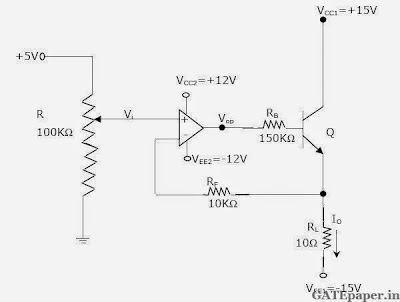### GATE 2002 ECE Video Solutions on Analog Circuits (Analog Electronics)

1. In a negative feedback amplifier using voltage-series (Voltage sampling and series mixing) feedback,
a. Ri decreases and Ro decreases
b. Ri decreases and Ro increases
c. Ri increases and Ro decreases
d. Ri increases and Ro increases

2. A 741 OP-AMP has a gain-bandwidth product of 1 MHz. A non-inverting amplifier using this OP-AMP and having a voltage gain of 20 dB will exhibit a –3 dB bandwidth of
a. 50 kHz
b. 100 kHz
c. 100/17 kHz
d. 1000/7.07 kHz

3. Three identical RC coupled transistor amplifiers are cascaded. If each of the amplifiers has a frequency response as shown in the figure, the overall frequency response is as given in4. The circuit in the figure employs positive feedback and is intended to generate sinusoidal oscillations. If at a frequency fo, β(f) = Vf(f)/Vo(f) = 1/6 ∟0o, then to sustain oscillations at this frequencya. R2 = 6R1
b. R2 = 5R1
c. R2 = R1/6
d. R2 = R1/5

5. An amplifier using an OP-AMP with a slew rate SR = 1 V/µsec has a gain of 40 dB. If this amplifier has to faithfully amplify sinusoidal signals form dc to 20 kHz without any slew rate induced distortion, then the input signal level must not exceed
a. 795 mV
b. 395 mV
c. 79.5 mV
d. 39.5 mV

6. The voltage gain Av = Vo/Vi of the JFET amplifier shown in the figure is IDSS = 10 mA, VP = 5 volts. Assume C1, C2 and Cs to be very large.a. + 16
b. – 16
c. + 8
d. – 6

7. A triangular voltage waveform Vi(t) is applied at the input to the circuit shown. Assume the diodes to be ideal.a. Determine the output Vo(t)
b. Neatly sketch the output waveform superimposed on the input Vi(t) and label the key points.

8. Below figure shown is a 2 – stage amplifier.The transistors Q1 and Q2 are identical with current gain β = 100, further βDC = βac = β. The zener diode Dz has a break down region and its dynamic resistance rz is zero. The capacitors C1 and C2 are large and provide negligible impedance at signal frequencies.
a. Identify the configuration in each of the amplifier stages. (i.e. whether CE, CC or CB)
b. Determine the quiescent quantities of IC1 and VC1.
c. Determine an expression for the voltage gain Vo/Vs and determine its value. (Assume VBE = 0.7 volts, ro = ∞ and thermal voltage VT = 25mV)
Solution:

9. Consider the circuit shown. Assume the operational amplifier is ideal.a. In which mode is the BJT operating (active or saturation or cutoff)? Justify your answer.
b. Obtain an expression relating the output current Io and the input voltage Vi.
c. Determine Io and Vop if Vi = 2 volt. Assume β = 99 and VBE = 0.7 volts.